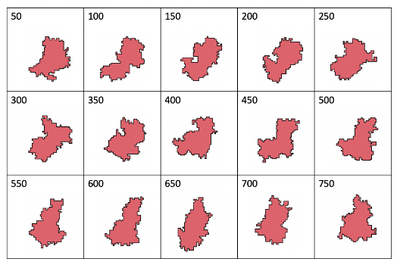# One CPM Cell

##### Persistent Identifier

Time sequence showing the changing shape of a CPM cell

## Introduction

Not all cell shapes are circular. In this example, a single cell undergoes a whole series of deformations resulting in the development of irregular cell shapes.

## Description

For a time sequence showing the shape of a CPM cell every $50$ time-steps the following parameters were used:

• Target area (VolumeConstraint) $a_0 = 400$,
• strength $\lambda_a = 1$,
• target perimeter (SurfaceConstraint) $p_0 = 120$,
• strength $\lambda_p = 1$,
• ConnectivityConstraint enabled,
• cell-medium Contact strength $= 2$,
• temperature $= 1$,
• yield $= 0.1$.

## Results

The cell continuously puts out protrusions in various directions.A time sequence showing the shape of a CPM cell every $50$ time steps.

## Model

Get this model via:

•  Download: OneCPMCell.xml
• XML Preview

<?xml version='1.0' encoding='UTF-8'?>
<MorpheusModel version="4">
<Description>
<Title>One CPM Cell</Title>
<Details>Full title:		One CPM Cell
Authors:		L. Edelstein-Keshet
Contributors:	Y. Xiao
Date:		02.05.2022
Model ID:		https://identifiers.org/morpheus/M2002
Reference:		L. Edelstein-Keshet: Mathematical Models in Cell Biology
Comment:		A time sequence showing the shape of a CPM cell every 50 time steps. The cell continuously puts out protrusions in various directions.</Details>
</Description>
<Space>
<Lattice class="square">
<Size symbol="l" value="150, 150, 0"/>
<BoundaryConditions>
<Condition type="constant" boundary="x"/>
<Condition type="constant" boundary="-x"/>
<Condition type="constant" boundary="y"/>
<Condition type="constant" boundary="-y"/>
</BoundaryConditions>
<NodeLength value="0.37"/>
<Neighborhood>
<Distance>1.5</Distance>
</Neighborhood>
</Lattice>
<SpaceSymbol symbol="space"/>
</Space>
<Time>
<StartTime value="0"/>
<StopTime symbol="end" value="750"/>
<TimeSymbol symbol="time"/>
<RandomSeed value="1227903"/>
</Time>
<CellTypes>
<CellType class="medium" name="medium"/>
<CellType class="biological" name="amoeba">
<VolumeConstraint target="400" strength="1"/>
<SurfaceConstraint target="120" strength="1" mode="surface"/>
<ConnectivityConstraint/>
</CellType>
</CellTypes>
<CPM>
<Interaction>
<Contact type1="amoeba" type2="amoeba" value="4"/>
<Contact type1="amoeba" type2="medium" value="2"/>
</Interaction>
<MonteCarloSampler stepper="edgelist">
<MCSDuration symbol="MCStime" value="0.1"/>
<Neighborhood>
<Order>2</Order>
</Neighborhood>
<MetropolisKinetics yield="0.1" temperature="1.0"/>
</MonteCarloSampler>
<ShapeSurface scaling="norm">
<Neighborhood>
<Distance>1.5</Distance>
</Neighborhood>
</ShapeSurface>
</CPM>
<CellPopulations>
<Population type="amoeba" size="1">
<InitCircle number-of-cells="1" mode="random">
</InitCircle>
</Population>
</CellPopulations>
<Analysis>
<Gnuplotter time-step="50" decorate="false">
<Terminal name="png" size="400 400 0"/>
<Plot>
<Cells opacity="0.65" min="0.0" max="10" value="cell.id">
<ColorMap>
<Color value="1" color="red"/>
<Color value="10" color="blue"/>
</ColorMap>
</Cells>
</Plot>
</Gnuplotter>
<ModelGraph format="svg" reduced="false" include-tags="#untagged"/>
</Analysis>
<Global/>
</MorpheusModel>